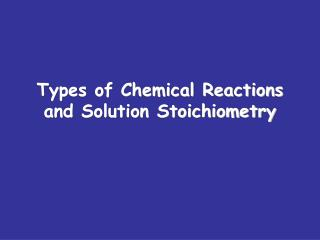DownloadDownload PresentationTypes of Chemical Reactions and Solution Stoichiometry

# Types of Chemical Reactions and Solution Stoichiometry

Download Presentation## Types of Chemical Reactions and Solution Stoichiometry

- - - - - - - - - - - - - - - - - - - - - - - - - - - E N D - - - - - - - - - - - - - - - - - - - - - - - - - - -
##### Presentation Transcript

1. Types of Chemical Reactionsand Solution Stoichiometry

2. An acid is a proton donor (H+ donor) A base is a proton acceptor ACID/BASE REACTION

3. ACID/BASE REACTIONStrong Acids Strong Bases • Sulfuric acid, H2SO4 • Nitric acid, HNO3 • Hydriodic acid, HI • Perchloric acid, HClO4 • Hydrobromic acid, HBr • Hydrochloric, HCl • Lithium hydroxide, LiOH • Rubidium hydroxide, RbOH • Potassium hydroxide, KOH • Sodium hydroxide, NaOH • Cesium hydroxide, CsOH • Calcium hydroxide, Ca(OH)2 • Barium hydroxide, Ba(OH)2 • Strontium hydroxide, Sr(OH)2

4. Strong Acid/Strong Base Reaction NaOH +HCl → NaCl + H2O Net Ionic H+ (aq)+ OH- (aq) → H2O ACID/BASE REACTION

5. Strong Acid/ Weak Base Reaction Generic Equation H+ (aq)+ B → BH+ (aq) ACID/BASE REACTION

6. Strong Acid/Weak Base HCl + NH3→ NH4 + (aq)+ Cl– (aq) Net ionic H+ (aq)+ NH3 → NH4 + (aq) ACID/BASE REACTION

7. Strong Acid/Weak Base Reaction H+ + CH3NH2→ CH3NH3+ CH3NH2 is a methylamine (base) ACID/BASE REACTION

8. STRONG BASE/WEAK ACID GENERIC EQUATION HB + OH-→ H2O + B- ACID/BASE REACTION

9. STRONG BASE/WEAK ACID HNO2 + KOH → H2O + KNO2 NET IONIC HNO2 + OH- (aq) → H2O + NO2- (aq) ACID/BASE REACTION

10. ACID/BASE STOICHIOMETRY • What volume of 0.500 M hydrochloric acid is needed to completely react with 30.0 ml of barium hydroxide?

11. ACID/BASE STOICHIOMETRY • 40.0 ml of nitric acid requires 100.0 ml of 0.500 M potassium hydroxide to neutralize it. What is the concentration of the original acid

12. ACID/BASE STOICHIOMETRY • 30.0 ml of 0.500 M ammonia is needed to neutralize 50 ml of hydrochloric acid? What was the molarity hydrochloric acid?

13. SOLUTION STOICHIOMETRY • Calculate the volume of 0.309 M sodium hydroxide required to completely react with • A. 15.9 ml of 0.190 M hydrofluoric acid? • B. 22.9 ml of 0.296 M perchloric acid?

14. ACID/BASE STOICHIOMETRY • What volume of 30.0 ml of 0.500 M potassium hydroxide is needed to completely neutralize 0.500 M acetic acid?